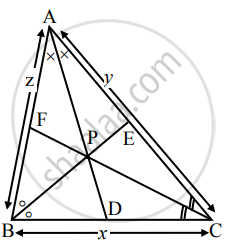# Prove that the angle bisectors of a triangle are concurrent - Mathematics and Statistics

Sum

Prove that the angle bisectors of a triangle are concurrent

#### SolutionConsider ∆ABC.

Let AD, BE and CF be the angle bisectors of ∆ABC. Let the lengths of sides AB, BC and CA be z, x and y respectively.

Let bar"a", bar"b", bar"c", bar"d", bar"e", bar"f" be the position vectors of points A, B, C, D, E, F respectively.

Now, point D divides BC internally in the ratio z : y

∴ bar"d" = ("y"bar"b" + "z"bar"c")/("y" + "z")  .......(i)

Point E divides CA internally in the ratio x : z

∴ bar"e" = ("z"bar"c" + xbar"a")/("z" + x)  .......(ii)

Point F divides AB internally in the ratio y : x

∴ bar"f" = (xbar"a" + ybar"b")/(x + "y")  .......(iii)

∴ From (i), (ii), (iii), we get

("y" + "z")bar"d" = "y"bar"b" + "z"bar"c"

("z" + x)bar"e" = "z"bar"c" + xbar"a"

(x+ "y")bar"f" = xbar"a" + "y"bar"b"

∴ ("y" + "z")bar"d" + xbar"a" = ("z" + x)bar"e" + "y"bar"b" = (x + "y")bar"f" + "z"bar"c"

= xbar"a" + y"b" + "z"bar"c"

∴ ((y + "z")bar"d" + xbar"a")/((y + "z") + x) =  (("z" + x)bar"e" + ybar"b")/(("z" + x) + y) = ((x + y)bar"f" + "z"bar"c")/((x + y) + "z") = (xbar"a" + y"b" + "z"bar"c")/(x + y + "z") = bar"p"    .......(say)

This shows that the point P whose position vector is p, lies on the three angle bisectors AD, BE and CF of ∆ABC dividing them in the ratios (y + z) : x, (z + x) : y and (x + y) : z respectively.

Hence, the three angle bisectors are concurrent.

Concept: Section Formula
Is there an error in this question or solution?